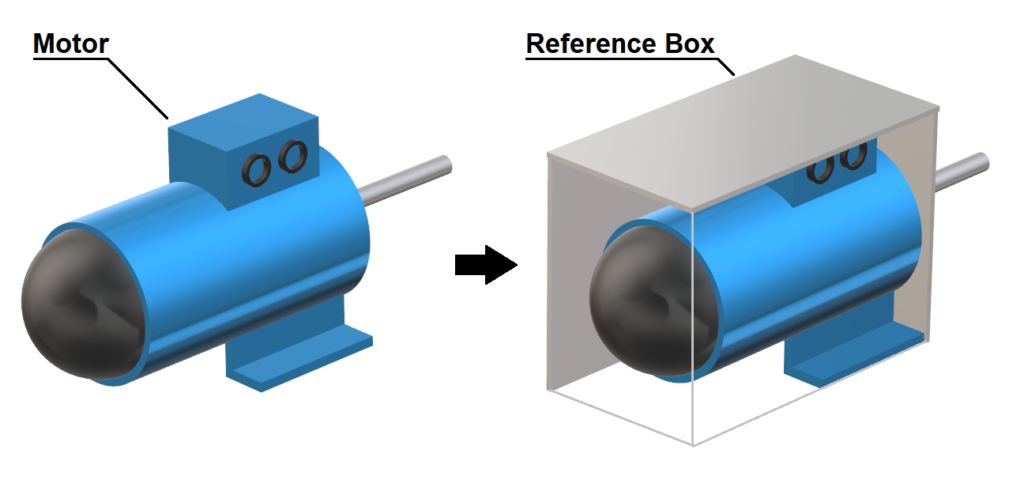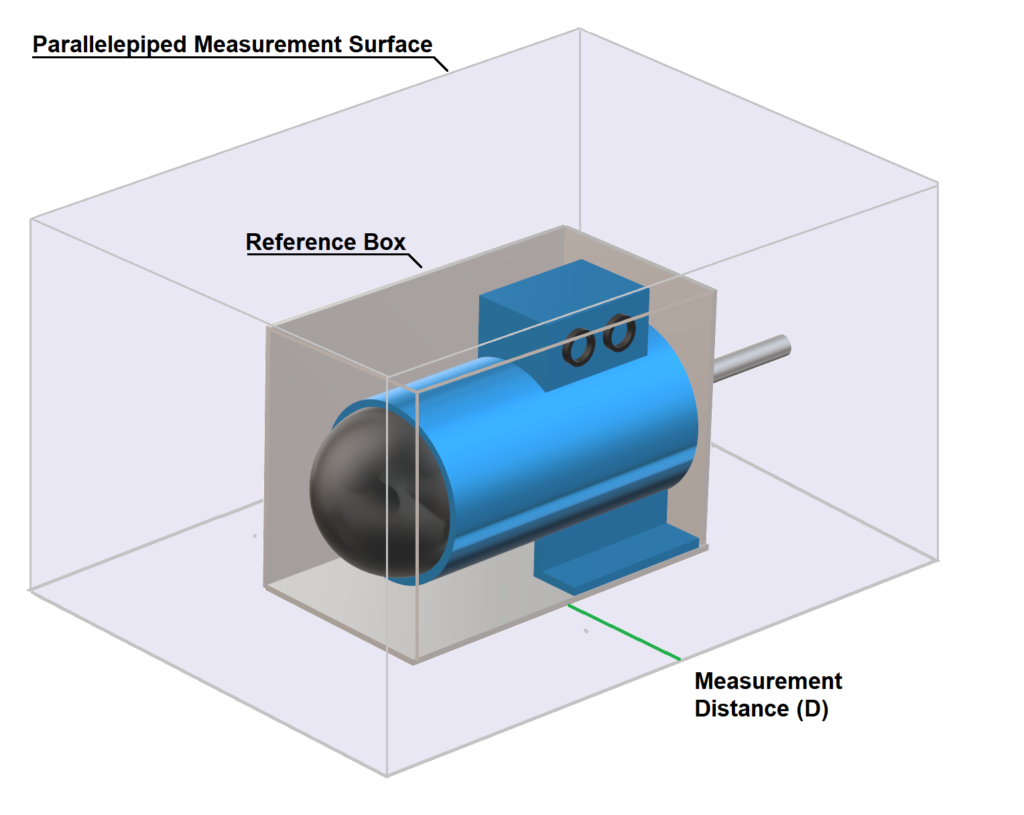# Sound Pressure to Sound Power Converter

## How do you calculate sound power from sound pressure?

In practice, engineers often deal with equipment sound levels in two measures – sound pressure levels and sound power levels. Where sound pressure level is a measure of the pressure amplitude of a sound wave, the sound power level is a measure of the energy of the sound wave. For more information on the physics of sound, the decibel scale and reference values, please visit the Physics Classroom.

It’s useful to understand the difference between sound pressure and sound power and to be able to estimate sound power from a sound pressure level. For example, if you know a source generates a sound pressure level of 85 dB(A) at 1 metre, with the dimensions of the source, you could determine the theoretical sound power level.

There are several standards that describe sound power estimation techniques including ISO 3746, ISO 3744 and ISO 3745. By applying certain assumptions, the standards’ methods can be used to approximate sound power from a sound pressure level.

For the sake of simplicity, the calculator below is based on the “measurement surface envelope” method defined in the ISO 3746 method. The applied assumptions include the following:

• There is one reflective plane (i.e. the ground); and,
• The sound pressure level is equivalent to the mean time-averaged sound pressure level (in other words, the average of noise measurements at different locations around the source).

## Step 1: Define the Reference Box

The first step is to define the reference box which is the smallest hypothetical rectangular box which encloses the source. See the example of a noisy motor in the figure below.## Step 2: Define the Measurement Surface

The second step is to define the measurement surface. Based on the source characteristic dimension criteria defined in the ISO standards, either the parallelepiped measurement surface or the hemispherical surface measurement is used.

The parallelepiped method involves defining a second, larger rectangular box with surfaces parallel to the reference box, positioned the measurement distance away from the source.

Whereas the hemispherical method involves defining a hemispherical measurement surface with a radius equivalent to the distance from the centre of the source to the measurement location. See examples of both in the figures below.## Step 3: Calculate the Sound Power Level

The sound power level is a function of the sound pressure level and the surface area of the measurement surface.

Use the calculators below for all 3 steps.

To calculate the attenuation of noise over distance using the inverse square law, see our sound inverse square law calculator.